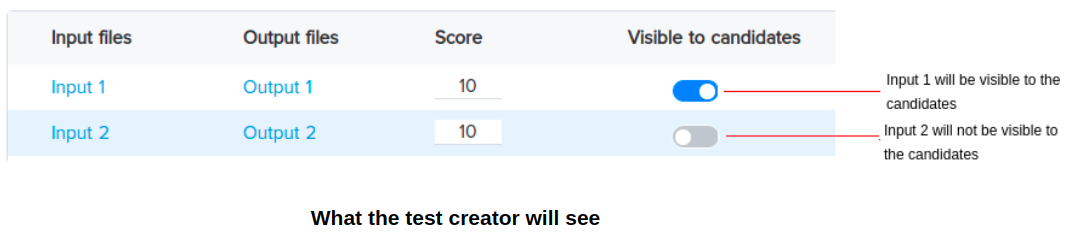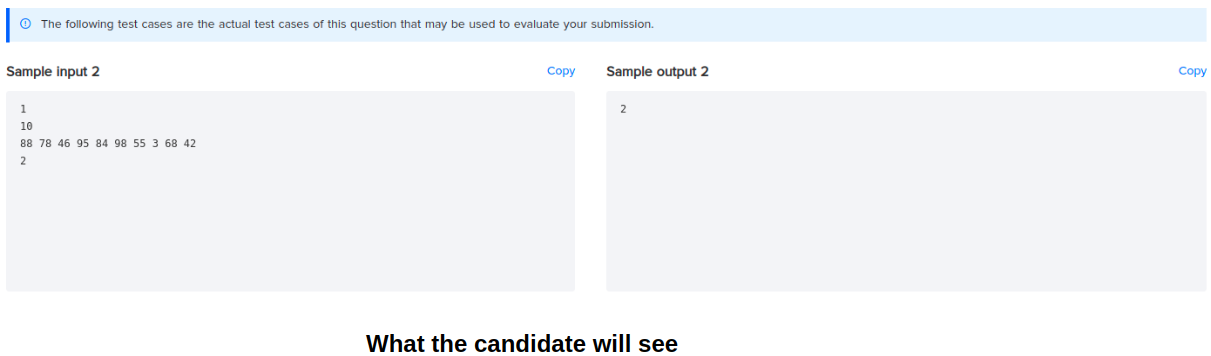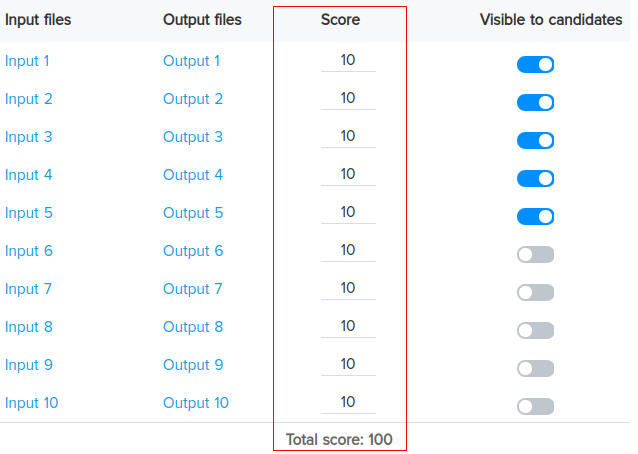Help Center

# Evaluating programming questions

Programming questions are automatically evaluated through HackerEarth’s evaluation engine. These questions are evaluated based on multiple test cases. A programming problem contains sample test cases and hidden test cases.

The sample test cases contain no scores because they are not considered while evaluating the code. Every problem has a certain number of hidden test cases. Based on the kind of problem, the number of test cases can range between 10 and 20. For example, if the answer to any problem is a single integer or a single character, then it must have multiple test cases.

For the purpose of this explanation, let’s consider that a problem has exactly 10 test cases. In this case:

• 5 test cases have small constraints.
• A brute force solution will work on these test cases.
• 2 of these 5 test cases are small (that is, readable). This is because recruiters have the option of making test cases visible to the candidate.• The remaining 5 test cases have large constraints
• Brute force will not pass, that is, the candidate’s submission will result in TLE if they apply a brute force solution.
• The total score of the hidden test cases is set to 100.
• Each test case is assigned a certain score. For the purpose of this explanation, let’s consider that there are 10 test cases that are assigned a score of 10 each.• You can customize the score for each test case based on your requirements.

How a candidate’s submission is evaluated

A candidate’s submission is evaluated based on the number of test cases that their solution passes. The generated score is the sum of the scores of the test cases that the candidate’s solution has passed.

For example, in this case, if the candidate’s solution passes 3 out of 10 test cases then their score will be 30/100.